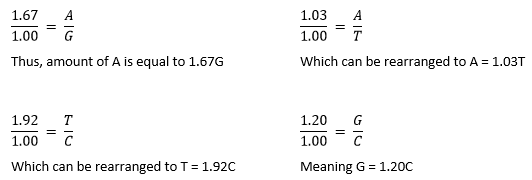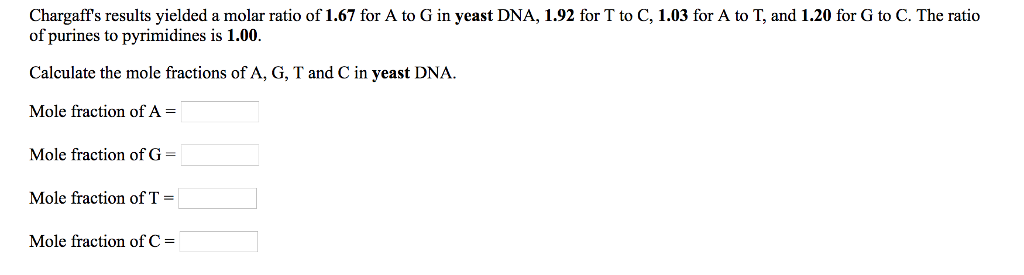# Problem: Chargaff's results yielded a molar ratio of 1.67 for A to G in yeast DNA, 1.92 for T to C, 1.03 for A to T, and 1.20 for G to C. The ratio of purines to pyrimidines is 1.00. Calculate the mole fractions of A, G, T, and C in yeast DNA.

🤓 Based on our data, we think this question is relevant for Professor Due-Goodwin's class at VANDERBILT.

###### FREE Expert Solution

The given ratios purine-pyrimidine ratio suggests that the given DNA sample is most likely double-stranded. However, non-equal amounts of A and T, as well as G and C (i.e. their ratios are not equal to 1), suggests otherwise. Thus, we simply proceed to systems of equations.Note that the ratio of purines to pyrimidines is 1, so A + G = T + C###### Problem Details

Chargaff's results yielded a molar ratio of 1.67 for A to G in yeast DNA, 1.92 for T to C, 1.03 for A to T, and 1.20 for G to C. The ratio of purines to pyrimidines is 1.00. Calculate the mole fractions of A, G, T, and C in yeast DNA.